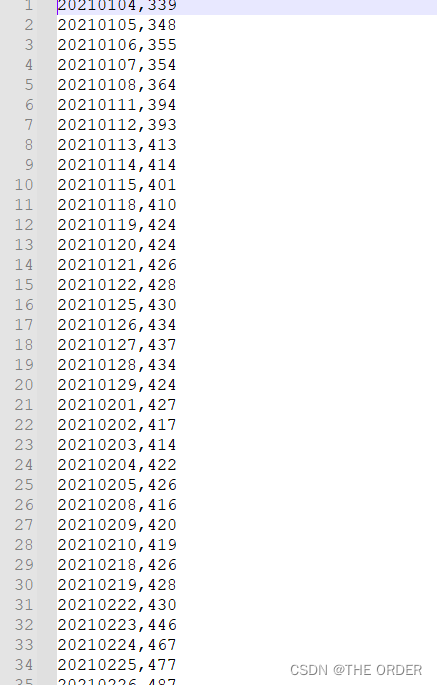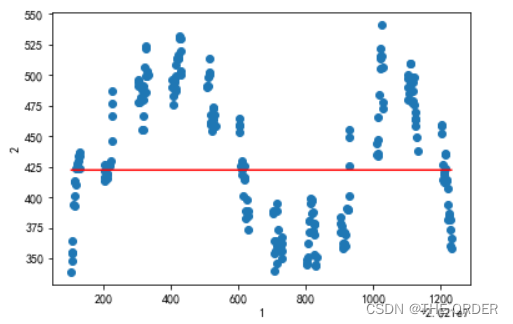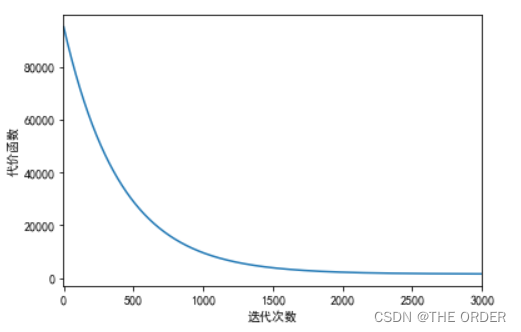# 1 环境准备

``````import numpy as np
import matplotlib.pyplot as pl
import matplotlib
matplotlib.rcParams['font.sans-serif']='SimHei'
matplotlib.rcParams['font.family']='sans-serif'
matplotlib.rcParams['axes.unicode_minus']=False
``````

# 2 读取文件方法设置

``````def loadDataset(filename):
X=[]
Y=[]
with open(filename,'rb') as f:
for idx,line in enumerate(f):
line=line.decode('utf-8').strip()
if not line:
continue

eles=line.split(',')

if idx==0:
numFea=len(eles)

eles=list(map(float,eles))#map&#x8FD4;&#x56DE;&#x4E00;&#x4E2A;&#x8FED;&#x4EE3;&#x5BF9;&#x8C61;

X.append(eles[:-1])
Y.append([eles[-1]])
return np.array(X),np.array(Y)
``````

# 3 预测值方法

def h(theta,X):
return np.dot(X,theta)

# 4 完成误差方法设计

def J(theta,X,Y):
return np.sum(np.dot((h(theta,X)-Y).T,(h(theta,X)-Y))/(2*m))

# 5 梯度下降方法

``````def bgd(alpha,maxloop,epsilon,X,Y):
m,n=X.shape

theta=np.zeros((2,1))

count=0
converged=False
error=np.inf
errors=[]
thetas={0:[theta[0,0]],1:[theta[1,0]]}

while count<=maxloop: if(converged): break count="count+1" temp1="theta[0,0]-alpha/m*(h(theta,X)-Y).sum()" temp2="theta[1,0]-alpha/m*(np.dot(X[:,1][:,np.newaxis].T,(h(theta,X)-Y))).sum()" #同步更新 theta[0,0]="temp1" theta[1,0]="temp2" thetas.append(temp1) thetas.append(temp2) error="J(theta,X,Y)" errors.append(error) if(error<epsilon): converged="True" return theta,errors,thetas < code></=maxloop:>
``````

# 6 读取文件``````X,Y=loadDataset('./data/price_diff.csv')
print(X.shape)
print(Y.shape)
``````

(243, 1)
(243, 1)

``````m,n=X.shape
X=np.concatenate((np.ones((m,1)),X),axis=1)
X.shape
``````

(243, 2)

# 7 模型参数设置

alpha=0.000000000000000003
maxloop=3000
epsilon=0.01
result=bgd(alpha,maxloop,epsilon,X,Y)
theta,errors,thetas=result

``````xCopy=X.copy()
xCopy.sort(0)
yHat=h(theta,xCopy)
xCopy[:,1].shape,yHat.shape,theta.shape
``````

((243,), (243, 1), (2, 1))

# 8 结果绘图

``````pl.xlabel(u'1')
pl.ylabel(u'2')
pl.plot(xCopy[:,1],yHat,color='red')
pl.scatter(X[:,1].flatten(),Y.T.flatten())
pl.show()
````````````pl.xlim(-1,3000)

pl.xlabel(u'&#x8FED;&#x4EE3;&#x6B21;&#x6570;')
pl.ylabel(u'&#x4EE3;&#x4EF7;&#x51FD;&#x6570;')
pl.plot(range(len(errors)),errors)
pl.show()
``````Original: https://blog.csdn.net/weixin_44498127/article/details/124547011
Author: THE ORDER
Title: 数据分析python，线性回归

## TensorFlow模型保存和加载

1. SavedModel格式 为了将训练好的机器学习模型部署到各个目标平台（如服务器、移动端、嵌入式设备和浏览器等），我们的第一步往往是将训练好的整个模型完整导出（序列化）为一系列标准格式的文件。在...